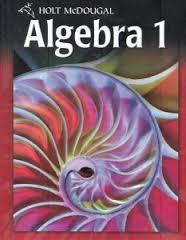1-7 HW Solving Absolute-value Equations

7 Questions | Total Attempts: 312Settings.

• 1.
#4, p. 57
• A.

X = 9; x = -9

• B.

X = 16; x = -16

• C.

No solution

• D.

All real numbers

• 2.
#6, p. 57
• A.

X = -5; x = 11

• B.

X = 1; x = 4

• C.

X = -6; x = 2

• D.

No solutions

• 3.
#9, p. 57
• A.

X = -3; x = -11

• B.

X = -1; x = 7

• C.

X = -6; x = 2

• D.

No solutions

• 4.
#16, p. 57
• A.

X = -5; x = 7

• B.

X = -6, x = 6

• C.

X = -10; x = 2

• D.

No solutions

• 5.
#2, p. 57
• A.

-4 and 4

• B.

-6 and 6

• C.

-10 and 2

• D.

-14 and 4

Related TopicsBack to top